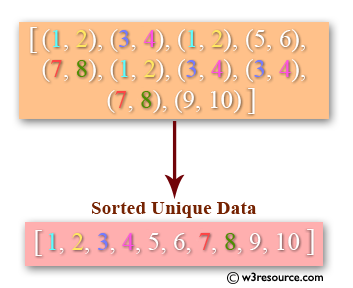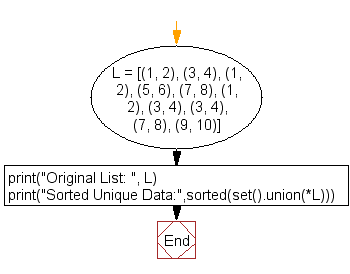﻿ Python: Convert a pair of values into a sorted unique array - w3resource# Python: Convert a pair of values into a sorted unique array

## Python List: Exercise - 45 with Solution

Write a Python program to convert a pair of values into a sorted unique array.Sample Solution:-

Python Code:

``````L = [(1, 2), (3, 4), (1, 2), (5, 6), (7, 8), (1, 2), (3, 4), (3, 4),
(7, 8), (9, 10)]
print("Original List: ", L)
print("Sorted Unique Data:",sorted(set().union(*L)))
```
```

Sample Output:

```Original List:  [(1, 2), (3, 4), (1, 2), (5, 6), (7, 8), (1, 2), (3, 4), (3, 4), (7, 8), (9, 10)]
Sorted Unique Data: [1, 2, 3, 4, 5, 6, 7, 8, 9, 10]
```

Flowchart:## Visualize Python code execution:

The following tool visualize what the computer is doing step-by-step as it executes the said program:

Python Code Editor:

Have another way to solve this solution? Contribute your code (and comments) through Disqus.

What is the difficulty level of this exercise?

Test your Python skills with w3resource's quiz

﻿

## Python: Tips of the Day

Floor Division:

When we speak of division we normally mean (/) float division operator, this will give a precise result in float format with decimals.

For a rounded integer result there is (//) floor division operator in Python. Floor division will only give integer results that are round numbers.

```print(1000 // 300)
print(1000 / 300)```

Output:

```3
3.3333333333333335```